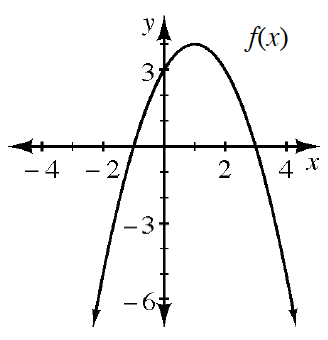### Home > AC > Chapter 12 > Lesson 12.2.1 > Problem12-40

12-40.Find the equation of $f(x)$ graphed at right.

Find the $x$-intercepts.
Their $x$-coordinates are roots of the equation.

See the homework help for problems 11-13 and 11-69.

Use the eTool below to help you find the equation for each function.
Click on the link at right for the full eTool version: 12-40 HW eTool